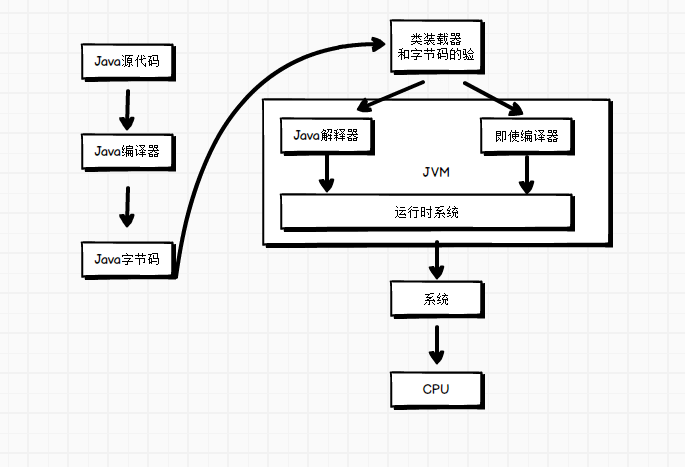程序如何运行的

   public static void main(String[] args) {
int i=17;
int j=5;
int sum=i+j;
System.out.println(sum);
}



CPU能做什么

1. 把15+5进行拆解就是 7+5=12，发现5+5有进位10；
2. 利用进位的十位与10+10 =20
3. 再把两个的和相加，20+2=22 ，没有再进位，运算结束。

	static int add(int i, int j){
if(j == 0)
return i;
int sum = i ^ j;//得到个位相加
int carry = (i & j) << 1;//得到进位相加
}


Java代码最终的编译结果(本文完)